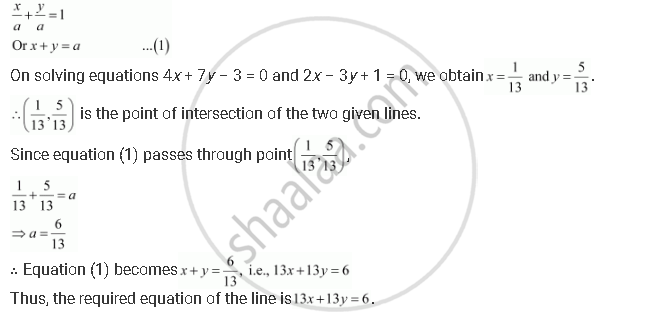CBSE (Arts) Class 11CBSE
Share

# Find the Equation of the Line Passing Through the Point of Intersection of the Lines 4x + 7y – 3 = 0 and 2x – 3y + 1 = 0 that Has Equal Intercepts on the Axes. - CBSE (Arts) Class 11 - Mathematics

ConceptGeneral Equation of a Line

#### Question

Find the equation of the line passing through the point of intersection of the lines 4x + 7y – 3 = 0 and 2x– 3y + 1 = 0 that has equal intercepts on the axes.

#### Solution

Let the equation of the line having equal intercepts on the axes beIs there an error in this question or solution?

#### Video TutorialsVIEW ALL 

Solution Find the Equation of the Line Passing Through the Point of Intersection of the Lines 4x + 7y – 3 = 0 and 2x – 3y + 1 = 0 that Has Equal Intercepts on the Axes. Concept: General Equation of a Line.
S